# Triangle radians

The size of two internal angles of a triangle ABC are α=6/18π and β=7/18π.

Calculate the size of the third angle.

Result

γ =  0.873 rad

#### Solution:Leave us a comment of example and its solution (i.e. if it is still somewhat unclear...):

Showing 0 comments:Be the first to comment!#### To solve this example are needed these knowledge from mathematics:

See also our trigonometric triangle calculator.

## Next similar examples:

1. It is rectangular?Size of two of the angles in a triangle are: α=110°, β=40°. Is it a right triangle?
2. AnglesThe triangle is one outer angle 158°54' and one internal angle 148°. Calculate the other internal angles of a triangle.
3. Triangle P2Can triangle have two right angles?
4. 3-bracket 3Two angles in a triangle are 90° and 60°. Has triangle at least two equal sides?
5. TrianglesEquilateral triangle with side 40 cm has the same perimeter as an isosceles triangle with arm of 45 cm. Calculate the base x of an isosceles triangle.
6. Isosceles triangle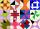Calculate the perimeter of isosceles triangle with arm length 26 cm and base length of 21 cm.
7. Area of RT 2Calculate the area of right triangle whose legs have a length 5.8 cm and 5.8 cm.
8. SimilarityAre two right triangles similar to each other if the first one has a acute angle 70° and second one has acute angle 20°?
9. 3-bracketMay be the largest angle in the triangle less than 20°?
10. 3-bracket 2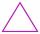May be the smallest angle in the triangle greater than 40°?
11. QuizQ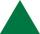An isosceles triangle has two sides of length 7 km and 39 km. How long is a third side?
12. TriangleCan be rectangular triangle equilateral?
13. Juice boxThe juice box has a volume of 200ml with its base is an isosceles triangle with sides a = 4,5cm and a height of 3,4cm. How tall is the box?
14. 7 triangle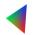The triangle area is 26.7 cm2. Determine the side length l if appropriate height hl = 45.3 cm.
15. Triangle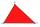For how many integer values of x can 16, 15 and x be the lengths of the sides of triangle?
16. Octagon from rectangleFrom tablecloth rectangular shape with dimensions of 4 dm and 8 dm we cuts down the corners in the shape of isosceles triangles. It thus formed an octagon with area 26 dm2. How many dm2 we cuts down?
17. MidpointsTriangle whose sides are midpoints of sides of triangle ABC has a perimeter 45. How long is perimeter of triangle ABC?